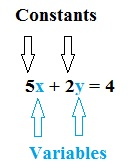# A List of Common and Uncommon Types of Variables

Standard

## A List of Common and Uncommon Types of VariablesWhile a “variable” in algebra really just means one thing–an unknown value–you’ll come across dozens of types of variables in statistics. Some are used more than others. For example, you’ll be much more likely to come across continuous variables than you would dummy variables.

## Common Types of Variables

• Categorical variable: variables than can be put into categories. For example, the category “Toothpaste Brands” might contain the variables Colgate and Aquafresh.
• Confounding variable: extra variables that have a hidden effect on your experimental results.
• Continuous variable: a variable with infinite number of values, like “time” or “weight”.
• Control variable: a factor in an experiment which must be held constant. For example, in an experiment to determine whether light makes plants grow faster, you would have to control for soil quality and water.
• Dependent variable: the outcome of an experiment. As you change the independent variable, you watch what happens to the dependent variable.
• Discrete variable: a variable that can only take on a certain number of values. For example, “number of cars in a parking lot” is discrete because a car park can only hold so many cars.
• Independent variable: a variable that is not affected by anything that you, the researcher, does. Usually plotted on the x-axis.
• measurement variable has a number associated with it. It’s an “amount” of something, or a”number” of something.
• Nominal variable: another name for categorical variable.
• Ordinal variable: similar to a categorical variable, but there is a clear order. For example, income levels of low, middle, and high could be considered ordinal.
• Qualitative variable: a broad category for any variable that can’t be counted (i.e. has no numerical value). Nominal and ordinal variables fall under this umbrella term.
• Quantitative variable: A broad category that includes any variable that can be counted, or has a numerical value associated with it. Examples of variables that fall into this category include discrete variables and ratio variables.
• Random variables are associated with random processes and give numbers to outcomes of random events.
• ranked variable is an ordinal variable; a variable where every data point can be put in order (1st, 2nd, 3rd, etc.).
• Ratio variables: similar to interval variables, but has a meaningful zero.

## Less Common Types of Variables

• Active Variable: a variable that is manipulated by the researcher.
• Attribute variable: another name for a categorical variable (in statistical software) or a variable that isn’t manipulated (in design of experiments).
• Binary variable: a variable that can only take on two values, usually 0/1. Could also be yes/no, tall/short or some other two-variable combination.
• Collider Variable: a variable represented by a node on a causal graph that has paths pointing in as well as out.
• Covariate variable: similar to an independent variable, it has an effect on the dependent variable but is usually not the variable of interest. See also: Noncomitant variable.
• Criterion variable: another name for a dependent variable, when the variable is used in non-experimental situations.
• Dichotomous variable: Another name for a binary variable.
• Dummy Variables: used in regression analysis when you want to assign relationships to unconnected categorical variables. For example, if you had the categories “has dogs” and “owns a car” you might assign a 1 to mean “has dogs” and 0 to mean “owns a car.”
• Endogenous variable: similar to dependent variables, they are affected by other variables in the system. Used almost exclusively in econometrics.
• Exogenous variable: variables that affect others in the system.
• Explanatory Variable: a type of independent variable. When a variable is independent, it is not affected at all by any other variables. When a variable isn’t independent for certain, it’s an explanatory variable.
• Extraneous variables are any variables that you are not intentionally studying in your experiment or test.
• grouping variable (also called a coding variable, group variable or by variable) sorts data within data files into categories or groups.
• Identifier Variables: variables used to uniquely identify situations.
• Indicator variable: another name for a dummy variable.
• Interval variable: a meaningful measurement between two variables. Also sometimes used as another name for a continuous variable.
• Intervening variable: a variable that is used to explain the relationship between variables.
• Latent Variable: a hidden variable that can’t be measured or observed directly.
• Manifest variable: a variable that can be directly observed or measured.
• Manipulated variable: another name for independent variable.
• Mediating variable: variables that explain how the relationship between variables happens. For example, it could explain the difference between the predictor and criterion.
• Moderating variable: changes the strength of an effect between independent and dependent variables. For example, psychotherapy may reduce stress levels for women more than men, so sex moderates the effect between psychotherapy and stress levels.
• Nuisance Variable: an extraneous variable that increases variability overall.
• Observed Variable: a measured variable (usually used in SEM).
• Outcome variable: similar in meaning to a dependent variable, but used in a non-experimental study.
• Polychotomous variables: variables that can have more than two values.
• Predictor variable: similar in meaning to the independent variable, but used in regression and in non-experimental studies.
• Responding variable: an informal term for dependent variable, usually used in science fairs.
• Scale Variable: basically, another name for a measurement variable.
• Test Variable: another name for the Dependent Variable.
• Treatment variable: another name for independent variable.

Source:  http://www.statisticshowto.com/types-variables/

#### 1 comment:

1.I don't want to get hung up on my studies and spend all my energy on it, so I found this site www.freepaperwriter.com/ where there are experts to write work for me, and I got an A, I like the fact that I don't have to do anything